# Fourier Transforms

1 Introduction 2 Integral Transforms 3 Fourier Integral Theorem 4 Fourier Transforms And Its Properties 5 Convolution Theorem And Parseval’s Theorem 6 Fourier Sine And Cosine Transforms

FOURIER TRANSFORMS

1 INTRODUCTION

2 INTEGRAL TRANSFORMS

3 FOURIER INTEGRAL THEOREM

4 FOURIER TRANSFORMS AND ITS PROPERTIES

5 CONVOLUTION THEOREM AND PARSEVAL’S THEOREM

6 FOURIER SINE AND COSINE TRANSFORMS

1 Introduction

This unit starts with integral transforms and presents three well-known integral transforms, namely, Complex Fourier transform, Fourier sine transform, Fourier cosine transform and their inverse transforms. The concept of Fourier transforms will be introduced after deriving the Fourier Integral Theorem. The various properties of these transforms and many solved examples are provided in this chapter. Moreover, the applications of Fourier Transforms in partial differential equations are many and are not included here because it is a wide area and beyond the scope of the book.

2 Integral Transforms

The integral transform f(s) of a function f(x) is defined byif the integral exists and is denoted by I{f(x)}. Here, K(s,x) is called the kernel of the transform. The kernel is a known function of „s‟ and „x‟. The function f(x) is called the  inverse transform of f(s). By properly selecting the kernel in the definition of general integral transform, we get various integral transforms.

The following are some of the well-known transforms:

(i) Laplace Transform(ii) Fourier Transform(iii) Mellin Transform(iv) Hankel Transformwhere Jn(sx)   is the Bessel function of the first kind and order 'n'.

3 FOURIER INTEGRAL THEOREM

If f(x) is defined in the interval (-ℓ,ℓ), and the following conditions

(i) f(x) satisfies the Dirichlet‟s conditions in every interval (-ℓ,ℓ),which is known as the Fourier integral of f(x).

Note:

When f(x) satisfies the conditions stated above, equation (3) holds good at a point of continuity. But at a point of discontinuity, the value of the integral is (1/ 2) [f(x+0) + f(x-0)] as in the case of Fourier series.

Fourier sine and cosine Integrals

The Fourier integral of f(x) is given byWhen f(x) is an odd function, f(t) coslt is odd while f(t) sinlt is even. Then the first integral of (4) vanishes and, we getwhich is known as the Fourier sine integral.

Similarly, when f(x) is an even function, (4) takes the formwhich is known as the Fourier cosine integral.

Complex form of Fourier Integrals

The Fourier integral of f(x) is given bySince cos l(t –x) is an even function of l, we have by the property of definite integralsSimilarly, since sin l(t –x) is an odd function of l, we havewhich is the complex form of the Fourier integral.

4 Fourier Transforms and its properties

Fourier Transform

We know that the complex form of Fourier integral isThe function F(s), defined by (1), is called the Fourier Transform of f(x). The function f(x), as given by (2), is called the inverse Fourier Transform of F(s). The equation (2) is also referred to as the inversion formula.

Properties of Fourier Transforms

(1) Linearity Property

If  F(s) and G(s) are Fourier Transforms of f(x) and g(x) respectively, then

F{a f(x) + bg(x)} = a F(s) + bG(s),

where a and b are constants.= a F(s) + bG(s) i.e,

F{a f(x) + bg(x)} = a F(s) + bG(s)

(2) Shifting Property

(i)                If  F(s) is the complex Fourier Transform of f(x), then

F{f(x-a)} = eisa F(s).(ii) If F(s) is the complex Fourier Transform of f(x), then(3) Change of scale property

If F(s) is the complex Fourier transform of f(x), then

F{f(ax)} =1/a F(s/a), a ¹0.Put  ax = t, so that dx = dt/a.(4) Modulation theorem.

If F(s) is the complex Fourier transform of f(x),

Then  F{f(x) cosax} = ½{F(s+a) + F(s-a)}.(5) nth derivative of the Fourier Transform

If F(s) is the complex Fourier Transform of f(x),

Then F{xn f(x)} = (-i)n dn/dsn .F(s).(6) Fourier Transform of the derivatives of a function.

If  F(s) is the complex Fourier Transform of f(x),

Then, F{f-isF(s) if„(x)}f(x)®0as x=® ±¥.In general, the Fourier transform of the nth derivative of f(x) is given by

F{f n(x)} = (-is)n F(s),

provided-1 ‟the derivatives frst®„n±¥.   vanish   as   x

Property (7)

If F(s) is the complex Fourier Transform of f(x), then FProperty (8)

If F(s) is the complex Fourier transform of f(x),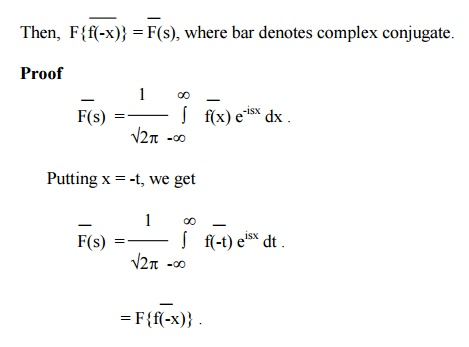Note:  If F{f(x)} = F(s), then

F{f(-x)} = F(-s).Example 1

Find the F.T of f(x) defined by

f(x) = 0       x<a

= 1    a<x<b

= 0    x>b.

The F.T of f(x) is given byExample 2

Find the F.T of f(x) = x  for  |x | =< a

= 0    for |x | > a.Example 3

Find the F.T of   f(x) = eiax ,  0 < x < 1

= 0 otherwise

The F.T of f(x) is given by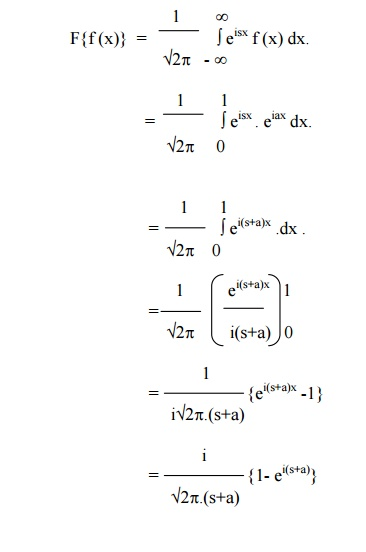Note:

If the F.T of f(x) is f(s), the function f(x) is called self-reciprocal. In the above example e -x 2/ 2 is self-reciprocal under F.T.

Example 5

Find the F.T of

f(x) = 1 for |x|<1.

= 0 for |x|>1.5 Convolution   Theorem . and   Parseval’s Theorem

The convolution of two functions f(x) and g(x) is defined asConvolution Theorem for Fourier Transforms.

The Fourier Transform of the convolution of f(x) and g(x) is the product of their Fourier Transforms,

i.e, F{f(x) * g(x)} = F{f(x).F{g(x)}.

Proof:

F{f(x) * g(x)} = F{(f*g)x)}Hence,  F{f(x) * g(x)} = F{f(x).F{g(x)}.

Parseval’s identity   for   Fourier   Transforms

If  F(s) is the F.T of f(x), thenProof:

By convolution theorem, we have

F{f(x) * g(x)} = F(s).G(s).

Therefore,  (f*g) (x) = F-1{F(s).G(s)}.Example 6

Find the F.T of f (x) = 1-|x|for |x|<1.

= 0    for |x|> 1Example 7

Find the F.T of f(x) if

f(x) =

= 1 for |x|<a

=  0 for |x|>a>0.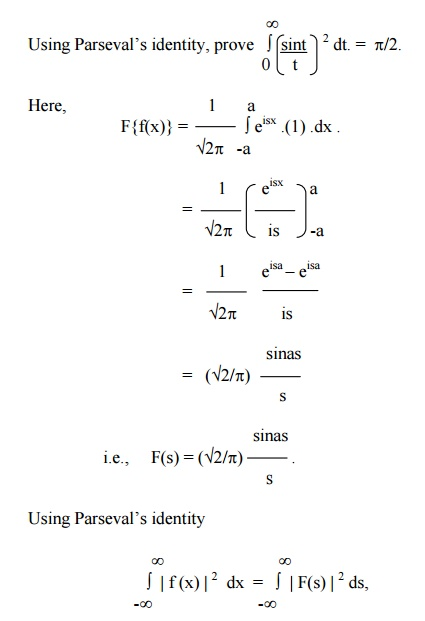6 Fourier sine and cosine transforms:

Fourier sine Transform

We know that the Fourier sine integral isThe function Fs(s), as defined by (1), is known as the Fourier sine transform of f(x). Also the function f(x), as given by (2),is called the Inverse Fourier sine transform of Fs(s) .

Fourier cosine transform

Similarly, it follows from the Fourier cosine integralThe function Fc(s), as defined by (3), is known as the Fourier cosine transform of f(x). Also the function f(x), as given by (4),is called the Inverse Fourier cosine transform of Fc(s) .

Properties of Fourier sine and cosine Transforms

If Fs(s) and Fc(s) are the Fourier sine and cosine transforms of f(x) respectively, the following properties and identities are true.

(1) Linearity property

Fs [a f(x) + b g(x) ] = a Fs { f(x) } + b Fs { g(x) }.

and  Fc [a f(x) + b g(x) ] = a Fc { f(x) } + b Fc { g(x) }.

(2) Change of scale property

Fs [ f(ax) ] = (1/a) Fs [ s/a ].

and   Fc [ f(ax) ] = (1/a) Fc [ s/a ].

(3) Modulation Theorem

i.           Fs [ f(x) sinax ] = (1/2) [ Fc (s-a) - Fc (s+a)].

ii.         Fs [ f(x) cosax ] = (1/2) [ Fs (s+a) + Fs (s-a)].

iii.      Fc[ f(x) cosax ] = (1/2) [ Fc (s+a) + Fc (s-a) ].

iv.      Fc[ f(x) sinax ] = (1/2) [ Fs (s+a) - Fs (s-a) ].

Proof

The Fourier sine transform of   f(x)sinax  is given by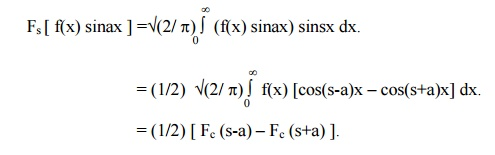Similarly, we can prove the results (ii), (iii) & (iv).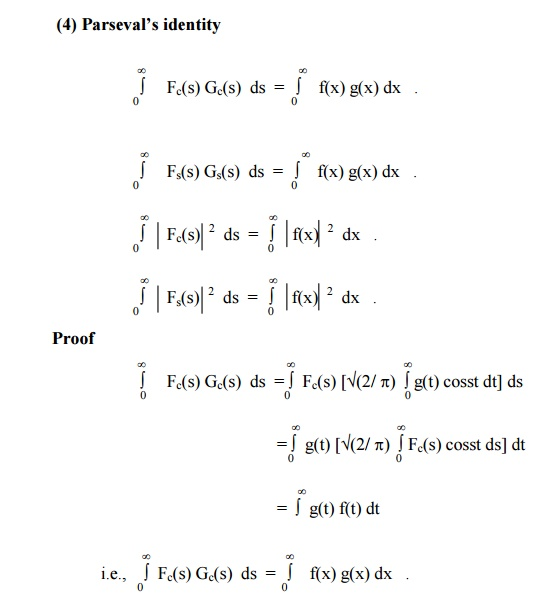Similarly, we can prove the second identity and the other identities follow by setting g(x) = f(x) in the first identity.

Property (5)

If  Fs(s) and Fc(s) are the Fourier sine and cosine transforms of f(x) respectively, then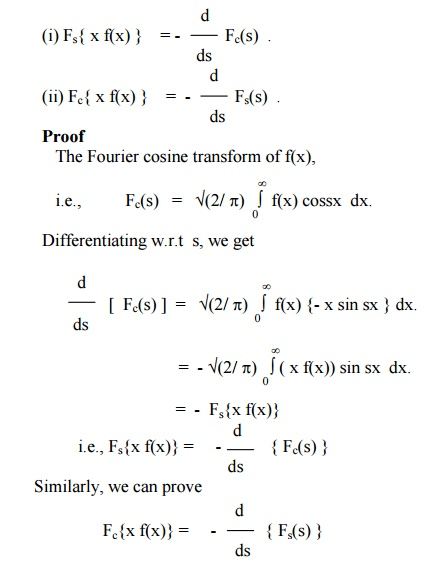Example 8

Find the Fourier sine and cosine transforms of e-ax and hence deduce the inversion formula.

The Fourier sine transform of  f(x) is given by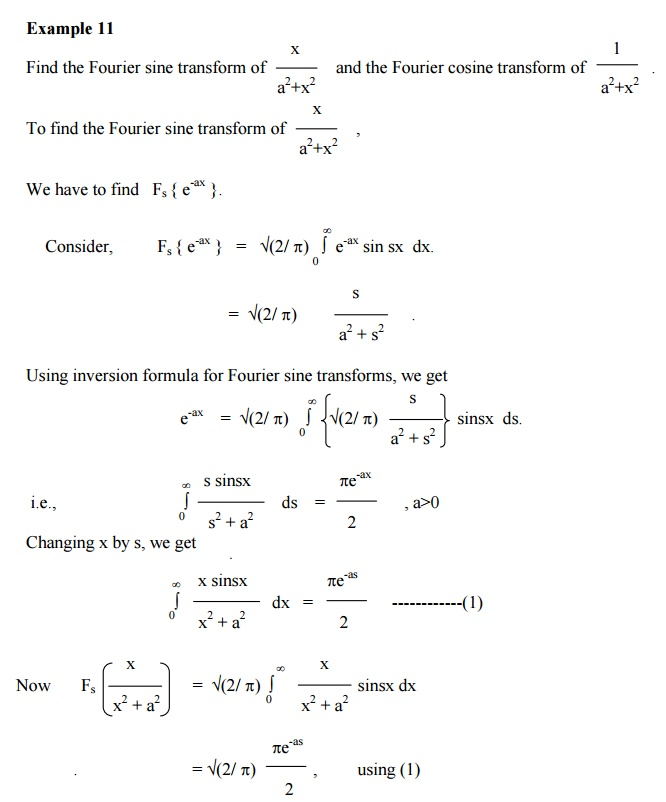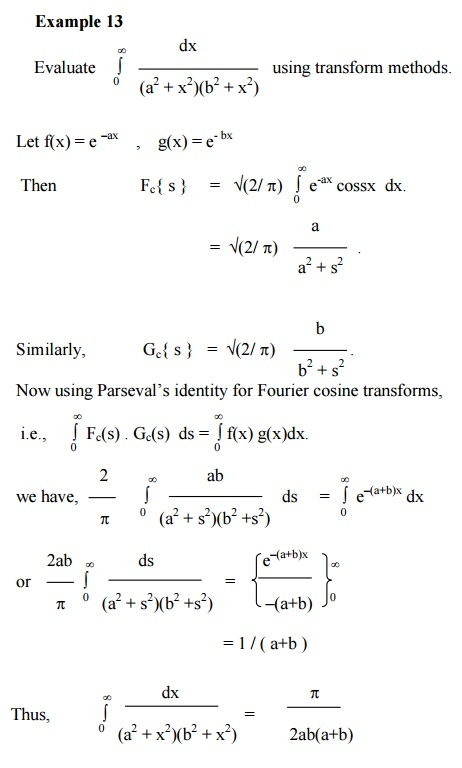Study Material, Lecturing Notes, Assignment, Reference, Wiki description explanation, brief detail
Mathematics (maths) : Fourier Transforms : Fourier Transforms |